Operations Research - Linear Programming - Graphical Statement and Solutions

by

Elmer G. Wiens

Egwald's popular web pages are provided without cost to users.
Please show your support by joining Egwald Web Services as a Facebook Fan:Follow Elmer Wiens on Twitter:The Primal and Dual Linear Programming Problems.

Linear programming problems come in pairs — a primal linear program (P) and an associated dual linear program (D). The linear objective function and the linear constraints of primal and dual programs of the linear programming problem are related in a specific way. I illustrate the mathematical statement of a linear programming problem with the following example.

 Primal Linear Program Maximize the Objective Function (P) P = 15 x1 + 10 x2 subject to L1: 0.25 x1 + 1.0 x2 <= 65 L2: 1.25 x1 + 0.5 x2 <= 90       x1 >= 0; x2 >= 0 Dual Linear Program Minimize the Objective Function (D) D = 65 y1 + 90 y2 subject to G1: 0.25 y1 + 1.25 y2 >= 15 G2: 1.0 y1 + 0.5 y2 >= 10       y1 >= 0; y2 >= 0

The following diagrams help one to visualize the primal and dual problems and suggest a way of solving linear programming problems. The area in yellow, called the feasible set, represents values of x1 and x2, (or y1 and y2), that satisfy the constraints L1 and L2, (or G1 and G2). Such vectors x = (x1, x2) (or y = (y1, y2) are called feasible vectors for the primal program (or dual program).

The lines P', P, and P'' in the primal diagram represent the objective function for different values of P, (988.89, 1288.89, 1588.89). Where P = 1288.89, the objective function (P) intersects the feasible set only at the point (x1, x2) = (51.111, 52.222). Here P attains its maximum value on the feasible set.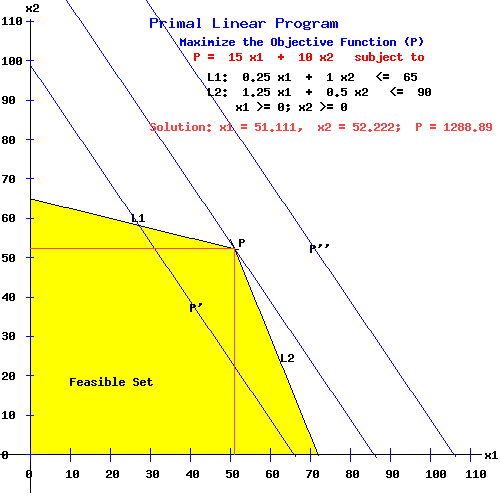The points (0,0 ), (72, 0), (51.111, 52.222), and (0, 65) are called the vertices of the feasible set. An optimal program obtains at a vertex of the feasible set.

Similarly, in the dual diagram where D = 1288.89, the objective function (D) intersects the feasible set only at the point (y1, y2) = (4.444, 11.111). Here D attains its minimum on the feasible set.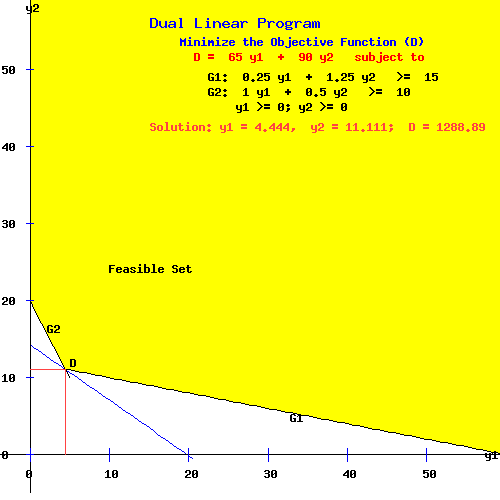Notes:

1. In the primal problem, the vector (x1, x2) = (51.111, 52.222) is the optimal primal program. For any other point in the feasible set, like (x'1, x'2) = (40, 40), the value of the objective function is less than the value of the objective function at the optimal program. i.e.,

15 x'1 + 10 x'2 = 15*40 + 10*40 = 1000 < 1288.89 = 15*51.111 + 10*52.222 = P

2. In the dual problem, the vector (y1, y2) = (4.444, 11.111) is the optimal dual program. For any other point in the feasible set, like (y'1, y'2) = (20, 20), the value of the objective function is greater than the value of the objective function at the optimal program. i.e.,

D = 1288.89 = 65*4.444 + 90*11.111 < 3100 = 65*20 + 90*20 = 65 y'1 + 90 y'2

Extreme Points and Basic Solutions.

The next diagram repeats the primal diagram above, but from a longer perspective. The primal problem has an extreme point at the intersection of any two constraints, including the non-negativity constraints. The six extreme points are (0, 180), (0, 65), (0, 0), (72, 0), (260, 0), and (51.111, 52.222). Each extreme point is called a basic solution, but only (0, 65), 0, 0), (72, 0), and (51.111, 52.222) are basic feasible solutions. An optimum basic feasible solution for the primal problem maximizes the objective function P on the feasible set.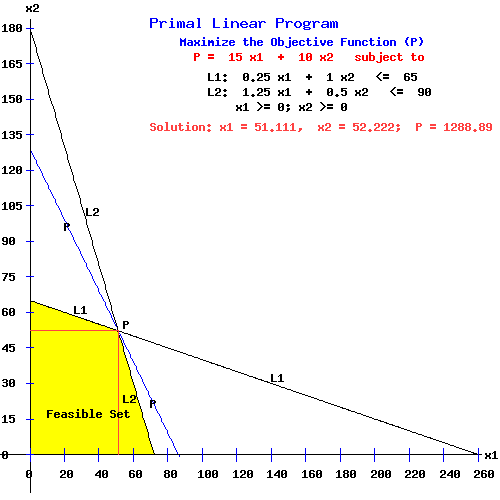The feasible set of a linear programming problem is called a convex set, because the feasible set includes the line connecting any two points in the feasible set, (including points on the boundary of the feasible set).

Unbounded and Unfeasible Problems.

The above linear programming problem is called feasible because the feasible sets of the primal and dual programs were non-empty. In other words, vectors x = (x1, x2) and y = (y1, y2) exist that satisfy the constraints (L1, L2) and (G1, G2).

In the next example, the primal problem is unbounded, and the dual problem is unfeasible.

 Primal Linear Program Maximize the Objective Function (P) P = 15 x1 + 10 x2 subject to L1: 0 x1 + 1.0 x2 <= 50 L2: -1.5 x1 + 1.0 x2 <= -20       x1 >= 0; x2 >= 0 Dual Linear Program Minimize the Objective Function (D) D = 50 y1 - 20 y2 subject to G1: 0 y1 - 1.5 y2 >= 15 G2: 1.0 y1 + 1.0 y2 >= 10       y1 >= 0; y2 >= 0

In the following diagram, the objective function P is unbounded since it intersects the feasible set for any large positive number = P.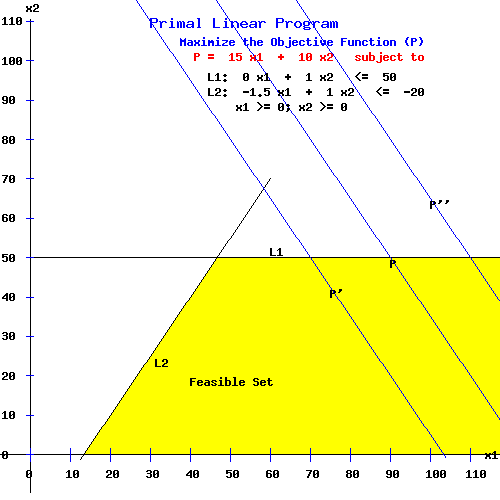The dual linear programming problem is unfeasible since the constraint G1 implies that y2 <= -10, contradicting the requirement that y2 >= 0.

If the primal program is unbounded, then the dual program is unfeasible, and vice versa. However, if the primal program is unfeasible, then the dual program is either unbounded or unfeasible, and vice versa.

The General Problem Statement.

 Primal Linear Program Maximize the Objective Function (P) P = c1 x1 + c2 x2 subject to L1: a11 x1 + a12 x2 <= b1 L2: a21 x1 + a22 x2 <= b2       x1 >= 0; x2 >= 0 Dual Linear Program Minimize the Objective Function (D) D = b1 y1 + b2 y2 subject to G1: a11 y1 + a21 y2 >= c1 G2: a12 y1 + a22 y2 >= c2       y1 >= 0; y2 >= 0

Using matrix and vector notation with the superscript T indicating the transpose of a matrix or vector, let :

 A = | a11 a12 | | a21 a22 | AT = | a11 a21 | | a12 a22 | x = | x1 || x2 | y = | y1 || y2 | b = | b1 || b2 | c = | c1 || c2 |

The primal / dual programming problem is stated conveniently as:

 Primal Linear Program Maximize the Objective Function (P) P = cT x subject to A x <= b, x >= 0 Dual Linear Program Minimize the Objective Function (D) D = yT b subject to yT A >= cT, y >= 0

This statement of the linear programming problem generalizes to A (m by n) matrix, x and b (1 by n), and y and c (1 by m) matrices.

Adding a Constraint.

Adding a constraint to the primal program adds a variable to the dual program. If the new constraint binds, the optimal programs shift, and the optimal value of each objective function is diminished.

 Primal Linear Program Maximize the Objective Function (P) P = 15 x1 + 10 x2 subject to L1: 0.25 x1 + 1.0 x2 <= 65 L2: 1.25 x1 + 0.5 x2 <= 90 L3: 1.0 x1 + 1.0 x2 <= 85       x1 >= 0; x2 >= 0 Dual Linear Program Minimize the Objective Function (D) D = 65 y1 + 90 y2 + 85 y3 subject to G1: 0.25 y1 + 1.25 y2 + 1.0 y3 >= 15 G2: 1.0 y1 + 0.5 y2 + 1.0 y3 >= 10       y1 >= 0; y2 >= 0; y3= 0

The primal optimal program, x = (x1, x2) = (63.333, 21.667), obtains at the intersection of the L2 and L3 constraint lines, with an optimal value for the objective function P = 1166.67.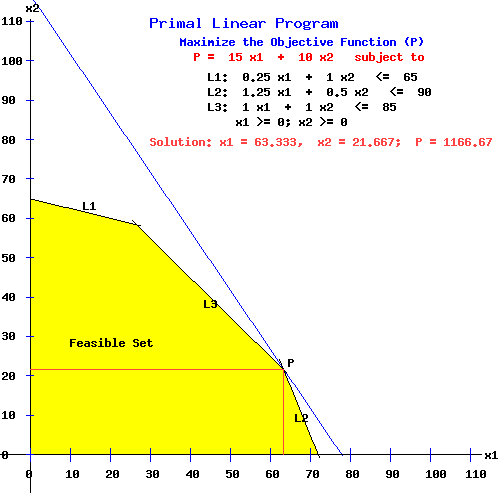This solution can be verified by using the online linear programming solver. If one proceeds this way, the results page displays as:

The L.P. Input Tableau
X1X2
Z001510
Z165-.25-1
Z290-1.25-.5
Z385-1-1
The L.P. Output Tableau
Y2Y3
Z1166.67-6.67-6.67
Y127.5-11.5
X163.33-1.330.67
X221.671.33-1.67

The dual optimal program is y = (y1, y2, y3) = (0, 6.67, 6.67), with an optimal value for the objective function:

D = 65 y1 + 90 y2 + 85 y3 = D = 65*0 + 90*6.6667 + 85*6.6667 = 1166.67.

The three dimensional dual graph shows this situation. In the diagram below, the objective function, D, and the constraints, G1 and G2, are planes in (Y1, Y2, Y3) space, while the G1 and G2 planes intersect along a line:

 D-Plane passing through (17.95, 0, 0), (0, 12.963, 0), and (0, 0, 13.73) when D = 1166.67 G1-plane passing through (60, 0, 0), (0, 12, 0), and (0, 0, 15) G2-plane passing through (10, 0, 0), (0, 20, 0), and (0, 0, 10) G1-plane & G2-plane intersect along the line passing through (4.44, 11.11, 0) and (0, 6.67, 6.67) With D = 1166.67, the D-plane intersects the dual feasible set only at the point (0, 6.67, 6.67); it intersects the G1 plane along the line through (1.84, 11.63, 0) and (0, 6.67, 6.67); and it intersects the G2 plane along the line through (5.51, 8.99, 0) and (0, 6.67, 6.67).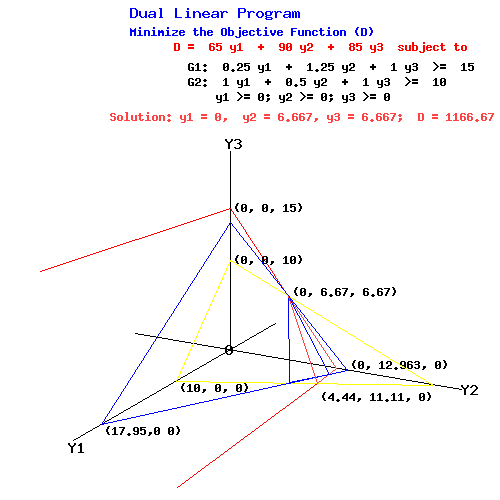Using the online linear programming solver is easier than using a 3-dimensional graph to find the solution when the problem has three or more variables.

Solving the dual problem directly.

One can obtain the solution to the dual problem directly using the online linear programming solver. Mulitply the dual objective function by -1 to change it to a maximization problem; similarily change the primal objective function to a minimization problem. Also, switch the x and y variable labels.

 Primal Linear Program Maximize the Objective Function (P) P = -65 x1 - 90 x2 - 85 x3 subject to G1: 0.25 x1 + 1.25 x2 + 1.0 x3 >= 15 G2: 1.0 x1 + 0.5 x2 + 1.0 x3 >= 10       x1 >= 0; x2 >= 0; x3 >= 0 Dual Linear Program Minimize the Objective Function (D) D = -15 y1 - 10 y2 subject to L1: 0.25 y1 + 1.0 y2 <= 65 L2: 1.25 y1 + 0.5 y2 <= 90 L3: 1.0 y1 + 1.0 y2 <= 85       y1 >= 0; y2 >= 0

Using the online linear programming solver, the results page displays as:

The L.P. Input Tableau
X1X2X3
Z00-65-90-85
Z115-.25-1.25-1
Z210-1-0.5-1
The L.P. Output Tableau
X1Y2Y1
Z-1166.67-27.5-21.67-63.33
X26.671-1.331.33
X36.67-1.51.67-0.67

Of course, solving the dual problem as the primal results in the same solution.

Slack Variables.

In a linear programming problem, each vector x determines a vector s = b - A x, the vector of slack variables for P; each vector y determines a vector tT = yT A - cT, the vector of slack variables for D. In the problem above:

 Primal Linear Program (P) L1: s1 = 65 - 0.25 x1 - 1.0 x2 L2: s2 = 90 - 1.25 x1 - 0.5 x2 L3: s3 = 85 - 1.0 x1 - 1.0 x2 Dual Linear Program (D) G1: t1 = 0.25 y1 + 1.25 y2 + 1.0 y3 - 15 G2: t2 = 1.0 y1 + 0.5 y2 + 1.0 y3 - 10

For x = (40, 30) the vector sT = (25, 25, 15); for y = (0, 10, 10) the vector tT = (7.5, 5).

For the optimal program x = (63.333, 21.667) the vector sT = (27.5, 0, 0); for the optimal program y = (0, 6.667, 6.667) the vector tT = (0, 0);

With slack variables, the constraints of this linear programming problem become the following systems of equations:

 Primal Program System of Equations: L1: 0.25 x1 + 1.0 x2 + 1 s1 + 0 s2 + 0 s3 = 65 L2: 1.25 x1 + 0.5 x2 + 0 s1 + 1 s2 + 0 s3 = 90 L3: 1.00 x1 + 1.0 x2 + 0 s1 + 0 s2 + 1 s3 = 85       x1 >= 0; x2 >= 0; s1 >= 0; s2 >= 0; s3 >= 0; Dual Program System of Equations: G1: 0.25 y1 + 1.25 y2 + 1.0 y3 + 1 t1 + 0 t2 = 15 G2: 1.00 y1 + 0.50 y2 + 1.0 y3 + 0 t1 + 1 t2 = 10       y1 >= 0; y2 >= 0; y3= 0; t1 >= 0; t2 >= 0;

The primal problem's system of equations has five variables and three equations. The diagram of this simultaneous system of linear equations for the constraints L1, L2, and L3 follows: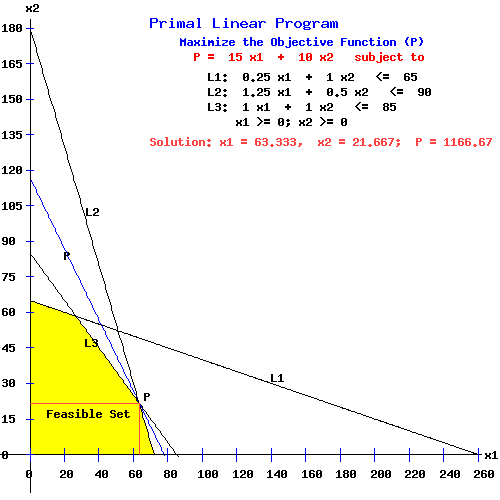In a basic solution for the primal problem's system of equations, the values of exactly 5 - 3 = 2 variables must be zero. For example, at the point (51.11, 52.22) at the intersection of the L1 and L2 equations, the system's variables take the values:

xT = (x1, x2) = (51.11, 52.22) and sT = (s1, s2, s3) = (0, -0, -18.33).

This basic solution is not a feasible solution because s3 = -18.33 is negative.

In a basic feasible solution for this system, the values of exactly 2 variables must be zero, the remaining 3 variables must be positive. For example, at the point (26.67, 58.33) at the intersection of the L1 and L3 equations, the system's variables take the values:

xT = (x1, x2) = (26.67, 58.33) and sT = (s1, s2, s3) = (0, 27.5, -0).

The optimum basic feasible solution for this system obtains at the point (63.33, 21.67) at the intersection of the L2 and L3 equations. Here the system's variables take the values:

xT = (x1, x2) = (63.33, 21.67) and sT = (s1, s2, s3) = (27.5, 0, 0).

The basic feasible solution at the origin deserves special mention. Here the system's variables take the values:

xT = (x1, x2) = (0, 0) and sT = (s1, s2, s3) = (65, 90, 85).

The primal simplex algorithm used to solve linear programming problems starts at the basic feasible solution at the origin, and proceeds to the optimum basic feasible solution along the vertices of the feasible set.

Let:

 A = | a11 a12 | | a21 a22 | | a31 a32 | x = | x1 || x2 | I3 = | 1 0 0 | | 0 1 0 | | 0 0 1 | s = | s1 || s2 || s3 | b = | b1 || b2 || b3 | y = | y1 || y2 || y3 | I2 = | 1 0 | | 0 1 | t = | t1 || t2 | c = | c1 || c2 |

With slack variables, the constraints of the linear programming problem can be stated in general as the systems of equations:

 A x + I3 s = b, x >= 0, s >= 0 yT A - tT I2 = cT, y >= 0, t >= 0

L.P. Solution Algorithms.

Many algorithms are available for solving linear programming problems. An early step in using a specific algorithm requires one to convert the mathematical statement of the linear programming problem to the standard form for that algorithm.

The standard form for the online solution algorithm permits one to have <=, >=, and = constraints in the specification of the feasible set for the primal problem. However, it insists that the RHS (right hand side) of the constraints be non-negative. i.e. the vector of coefficients (b1, b2, ... bn) >= 0.

Many text books introduce students to the simplex algorithm, a computational method that one can use to solve small l.p. problems "by hand," permitting one to learn a systematic method to compute a solution and to understand how the solution depends on the constraints and the objective function.

In the general linear programming problem, A is an (m by n) matrix, Im is the m dimensional identity matrix; x, t, and b are n-dimensional column vectors; In is the n dimensional identity matrix; and y, s and c are m-dimensional column vectors.

The specification of a linear programming problem is said to be in canonical form if all constraints are stated as <= constraints without slack variables s and t; it is said to be in a standard form if stated with slack variables s and t and all constraints are = constraints.

 Primal Linear Program Maximize the Objective Function (P) P = cT x subject to
 Dual Linear Program Minimize the Objective Function (D) D = yT b subject to
A x <= b, x >= 0 A x + Im s = b, x >= 0, s >= 0 yT A >= cT, y >= 0 yT A - tT In = cT, y >= 0, t >= 0

To convert the primal problem to canonical form, change any = constraints into a pair of <= and >= constraints. Change all >= constraints to <= constraints by muliplying both sides of the constraint by -1. When all constraints in the primal program are <= constraints, all constraints in the dual problem must be >= constraints.

If the linear programming problem has a variable xk that is unrestricted in sign, replace xk in each constraint with the difference of two new non-negative variables. i.e. xk = x'k - x''k; x'k >= 0, x''k >= 0.

(Note that absolute value expressions can be changed into a pair of <= and >= constraints by definition, i.e. |a| <= b implies that -b <= a <= b, and |a| >= b implies that either a >= b, or a <= -b.)

The standard form of the primal problem includes m simultaneous linear equations in n + m variables. Let the matrix A consist of the concatenation of matrices A and Im, i.e. A = [A | Im]. From linear algebra I know that a basic solution (a solution with exactly m non-zero variables) can be written as:

xB = B*b

where B is the inverse of a matrix, Å, formed by selecting m appropriate linearly independent columns of the matrix A.

Consequently, the number of possible basic solutions = (n + m)! / (n! * m!), the number of combinations of n + m variables taken m at a time.

For example, the system above with 2 primal variables and 3 slack variables has (2 + 3)! / (2! * 3!) = 10 basic solutions or extreme points.

Its A = [A | I3] matrix is:

 A = 0.25 1 1 0 0 1.25 0.5 0 1 0 1 1 0 0 1

If I select the columns of A corresponding to the variables x1, x2, and s2, the Å matrix and its inverse, B, are:

 Å = 0.25 1 1 1.25 0.5 0 1 1 0 B = -1.33 0 1.33 1.33 0 -0.33 1 1 -1.5

Since the vector bT = (65, 90, 85), the basic solution xB is:

xB = (x1, x2, s2) = B*b = (26.6667, 58.3333, 27.5000),

the basic solution at the intersection of the L1 and L3 constraints.

Complementary Necessary and Sufficient Conditions.

If the vectors x, s, y, t satisfy the primal and dual program constraints, and the complementary slackness conditions:

 Primal Linear Program
 Dual Linear Program
A x + s = b, x >= 0, s >= 0 yT A - tT = cT, y >= 0, t >= 0
Complementary Slackness
yi*si = 0, i = (1, ..., m}
--> yTs = 0
xj*tj = 0, j = (1, ..., n}
--> tTx = 0

then x (x, s) is an optimal primal program, and y (y, t) is an optimal dual program.

If x and y are optimal programs, then for any other pair of feasible programs x and y:

cTx <= cTx = yTb <= yTb

On the Simplex Algorithm web page I present methods to solve linear programming problems by hand. While more sophisticated algorithms are used on computers, the simplex algorithm and primal simplex algorithm permit one to get a grasp of linear programming knowing just the basics of linear algebra. Furthermore, these algorithms were the first ones developed, and were an important step in the development of more sophisticated algorithms, like the dual simplex algorithm, and Karmarkar's algorithm of 1984.

Linear Programming References.

• Budnick, Frank S., Richard Mojena, and Thomas E. Vollmann. Principles of Operations Research for Management. Homewood: Irwin, 1977.
• Dantzig, G., A. Orden, and P. Wolfe. "The Generalized Simplex Method for Minimizing a Linear Form under Inequality Restraints." Pacific Journal of Mathematics. 8, (1955): 183-195.
• Dantzig, George B. Linear Programming and Extensions. Princeton: Princeton U P, 1963.
• Dorfman, R., P. Samuelson, and R. Solow. Linear Programming and Economics Analysis. New York: McGraw-Hill, 1958.
• Hadley, G. Linear Algebra. Reading, Mass.: Addison-Wesley, 1961.
• Hadley, G. Linear Programming. Reading, Mass.: Addison-Wesley, 1962.
• Hillier, Frederick S. and Gerald J. Lieberman. Operations Research.. 2nd ed. San Francisco: Holden-Day, 1974.
• Karlin, S. Mathematical Methods in the Theory of Games and Programming. Vols. I & II. Reading, Mass.: Addison-Wesley, 1959.
• Loomba, N. Paul. Linear Programming: An Introductory Analysis. New York: McGraw-Hill, 1964.
• Press, William H., Brian P. Flannery, Saul A. Teukolsky, and William T. Vetterling. Numerical Recipes: The Art of Scientific Computing. Cambridge: Cambridge UP, 1989.
• Saaty, Thomas L. Mathematical Methods of Operations Research. New York: McGraw-Hill, 1959.
• Sierksma, Gerard. Linear and Integer Programming: Theory and Practice. 2nd ed. New York: Marcel Dekker, 2002.
• Sposito, Vincent. Linear and Nonlinear Programming. Ames, Iowa: Iowa UP, 1975.
• Sposito, Vincent. Linear Programming with Statistical Applications. Ames, Iowa: Iowa UP, 1989.
• Strang, Gilbert. Linear Algebra and Its Applications.3rd ed. San Diego: Harcourt, 1988.
• Restrepo, Rodrigo A. Theory of Games and Programming: Mathematics Lecture Notes. Vancouver: University of B.C., 1967.
• Restrepo, Rodrigo A. Linear Programming and Complementarity. Vancouver: University of B.C., 1994.
• Taha, Hamdy A. Operations Research: An Introduction. 2nd ed. New York: MacMillan, 1976.
• Wagner, Harvey M. Principles of Management Science: With Applications to Executive Decisions. 2nd ed. Englewood Cliffs: Prentice-Hall, 1975.
• Wagner, Harvey M. Principles of Operations Research: With Applications to Managerial Decisions. 2nd ed. Englewood Cliffs: Prentice-Hall, 1975.
• Wu, Nesa, and Richard Coppins. Linear Programming and Extensions. New York: McGraw-Hill, 1981.Copyright © Elmer G. Wiens:   EgwaldWeb ServicesAll Rights Reserved.    Inquiries